Start typing, then use the up and down arrows to select an option from the list.# Clausius-Clapeyron Equation Concept 2

Jules Bruno
439views
1
now the classiest clapper on equation can be changed into different forms. The first time we're going to talk about is the linear form of it. We're gonna say we use this form of the equation when a plot of the natural law which is Ln of pressure versus inverse temperature is given. So if we talk about this we can look at it in terms of our graph here and any time we do a plot, remember a plot is always of Y versus X. Here Ry is L. N. P. Which is why it's on the y axis. And then inverse temperature just means one over T. That represents our X. So that's why it's here on the X axis. So we have inverse of the pressure and then we have inverse temperature one over T. Okay, basically we're gonna use the line form of the classes Clapper on equation to help us determine the entropy of vaporization which is delta H Vape. And that's connected to the idea of our slope intercept form of the straight line which is why equals mx plus B. So again, our why axis has L. N. P. R. X. Has won over team here with this information we can find our slope which equals rise over run which remember is change in your y divided by change in your ex here are linear form of the classes Clapper on equation becomes L. N. P equals negative delta H. Vape over R times one over T plus C. Again this is related to the slope intercept form of a straight line. So this would be Y equals M X plus B. M. Represents our slope which represents negative delta H. Vape. Over our now our itself remembers r gas constant, it's 8.314 with the units of jewels over moles times kelvin pressure here is P is our vapor pressure and it's in units of tour or millimeters of mercury. When we talk about our delta H A Vape, that's our entropy of vaporization here. It's customarily in jewels per mole. Sometimes you might see it in killer joules per mole but just make sure units match the units of our our in jewels. So that's why we tend to see delta H. A Vape in jewels. Now we can also say our temperature here is temperature in kelvin and then see is just the constant of a substance. Now again, when it comes to the linear form of the classes Clapper on equation. The most important part is this portion here in blue. This portion here helps us to link together the concept of slope and our entropy of vaporization. So keep that in mind when we're given the plot a substance where it's LMP versus inverse temperature and they're asking us to determine either the slope or the entropy of vaporization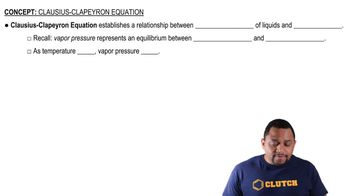00:59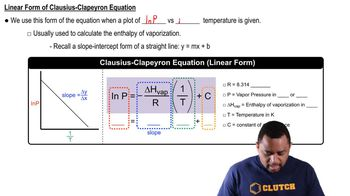02:57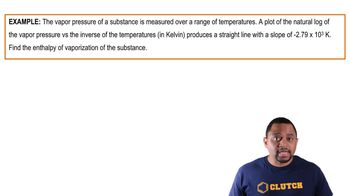01:37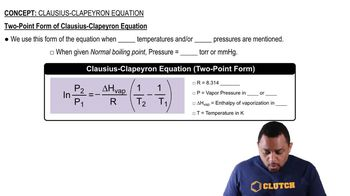01:13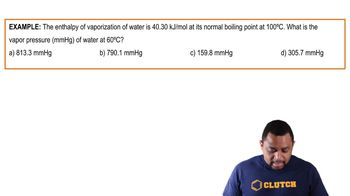03:37